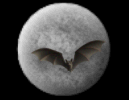Bigbang Forum Message Forums: Atm · Astrophotography · Blackholes · Blackholes2 · CCD · Celestron · Domes · Education Eyepieces · Meade · Misc. · God and Science · SETI · Software · UFO · XEphemBe the first pioneers to continue the Astronomy Discussions at our new Astronomy meeting place...The Space and Astronomy Agora String Theory As I Know It Forum List | Follow Ups | Post Message | Back to Thread Topics | In Response ToPosted by Richard Ruquist on May 9, 2005 17:21:20 UTC

Eric,

The original string theory developed by Max Green and Schwartz at CIT was not supersymmetric and was only for closed loops, which means a Boson-only theory. The equations are fairly simple. You can find them in the book on string theory Vol. I by Green, Schwartz and Witten.

It all comes down to a factor(z-26) where the expression is infinite except when z, the number of dimensions equals 26. That is essentially the theoretical proof that the universe contains 26 dimensions.

The original theory had several problems. It admitted tachyons, particles that exceed the speed of light, and it excluded fermions, the massive charged particles like quarks. So Max Green assumed supersymmetry, the existence of particles that cancel out the infinities at other values of z. His first solution was for z=10. That's the first one he published that made string theory what it is today.

Subsequently, 3 more z=10 solutions were found, another at z=9, z includes the time dimension BTW, and M theory by Witten had z=11, and Vafa theory had z=13, half way to 26. Who knows what the other half is for.

I have a model for dark matter based on string theory. In order to make fermions you need 9 space and 1 time dimension, but 6 of these dimensions are compactified. There is an experiment that simulates the creation of fermions or matter. A particle-anti-particle pair is produced at a point in z=10 space. But they fly off in opposite directions in z=3 space.

Strnagely the two particles have been shown to behave like they were still connected. My assumption is that they are connected in the z=6 compactified space by tiny particles called axions. The axions are within a string that connects the particle to the distant anti-particle.

But the connection is lost if either particle interacts with the environment. The axions then precipatate into space. In the big bang, the two oppositely charged particles eventually recombine (with others) with just a tiny fraction of the original number left over. That left over mass is the mass of the detectable universe. Dark matter in this model is the axion precipitate. As far as astronomers can tell, the invisible and undetectable dark matter mass density exceeds the detectable matter by an order of magnitude, and in fact makes up about 1/3 of the mass-equivalent energy density of the universe, the remainder being dark energy, also undetectable.

Dark energy in this way of thinking, comes from the two particles, the particle pair, actually being connected in all 22 compactified dimensions, assuming the universe is z=26.The axion comes from 6 of these compactified dimensions. It is likely, in my opinion, that dark energy is composed of particles associated with the remaining 16 compactified dimensions.

Richard